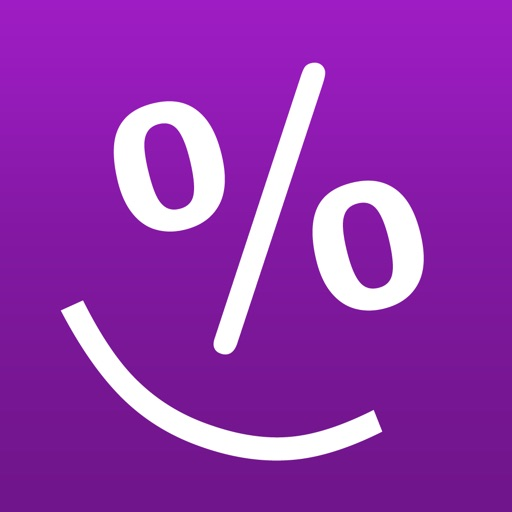Smart Percentage Calculator is a must-have app for everyday calculations - simple percentage calculations, percentage increase/decrease, calculating tips, sale price, percentage off, discounted price, and moreSmart Percentage Calculator

by Intemodino Group s.r.o.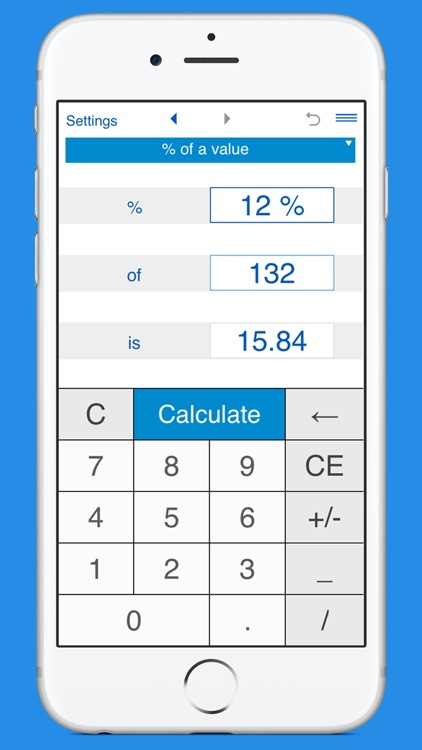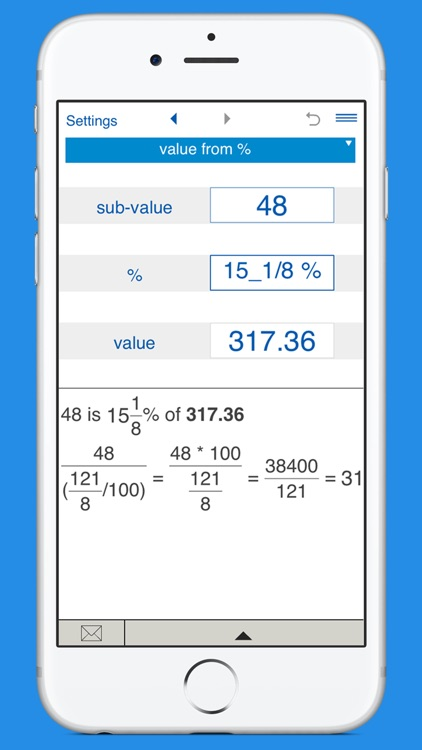Smart Percentage Calculator is a must-have app for everyday calculations - simple percentage calculations, percentage increase/decrease, calculating tips, sale price, percentage off, discounted price, and more.App Details

Version
2.1.0
Rating
(4)
Size
24Mb
Genre
Last updated
February 11, 2019
Release date
March 5, 2016

App Screenshots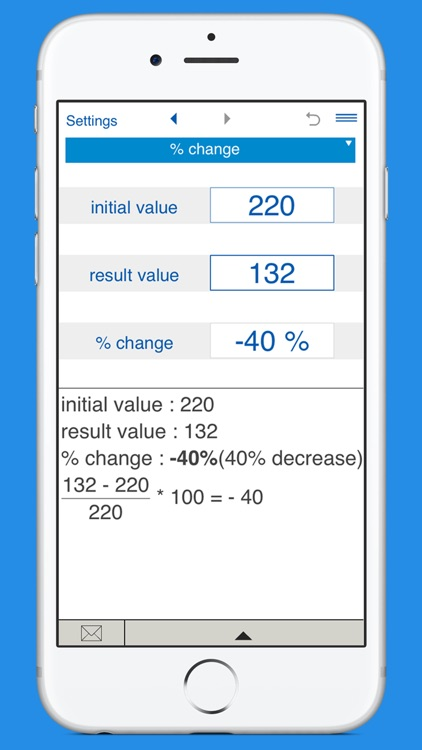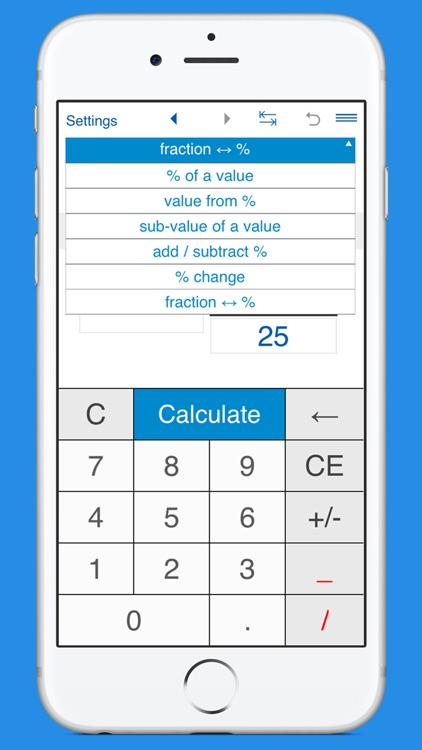App Store Description

Smart Percentage Calculator is a must-have app for everyday calculations - simple percentage calculations, percentage increase/decrease, calculating tips, sale price, percentage off, discounted price, and more.

Features:

• SIMPLE PERCENTAGE:
- finds the percentage of a value (what is 5% of 20?)
- finds the value from a percentage - finds the whole number when you know the percentage and the sub-value (if 4 is 30%, what is the whole number?)
- sub-value of a value - finds the proportion of one number to another in percentage terms (20 is what percent of 150?)

• ADD OR SUBTRACT A PERCENTAGE

• PERCENTAGE CHANGE
Finds the percentage change (increase/decrease) between two values.

• FRACTION TO PERCENTAGE
Converts fractions to percentages and percentages to fractions.

Smart Percentage Calculator makes calculations based on various inputs including values, sub-values, percentages, ratios, fractions, percentage changes, percentage increase/decrease.
If any two of values are entered, the calculator finds the third one.

Supports fractional values.

You can select the number of decimal places you want to display in results. By default, the app displays 2 decimal places.

History tape to view recent calculations (25 entries). Back and forward buttons lets you view your recent calculations.

Shares results and history via email.

Disclaimer:
AppAdvice does not own this application and only provides images and links contained in the iTunes Search API, to help our users find the best apps to download. If you are the developer of this app and would like your information removed, please send a request to [email protected] and your information will be removed.